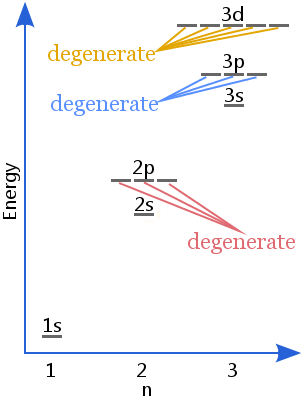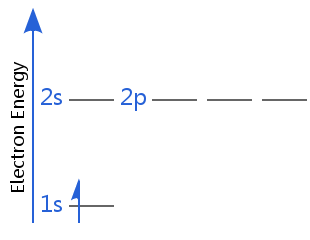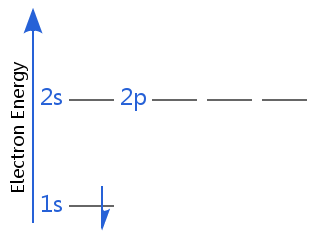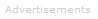# Definition of Degenerate

Degenerate is used in quantum mechanics to mean 'of equal energy.' It usually refers to electron energy levels or sublevels.For example, orbitals in the 2p sublevel are degenerate - in other words the 2px, 2py, and 2pz orbitals are equal in energy, as shown in the diagram.

Likewise, at a higher energy than 2p, the 3px, 3py, and 3pz orbitals are degenerate.

And, at a still higher energy, the 3dxy, 3dxz, 3dyz, 3dx2 - y2, and 3dz2 are degenerate.

The number of different states of equal energy is called the degree of degeneracy or just degeneracy.

The degeneracy of p orbitals is 3; the degeneracy of d orbitals is 5; the degeneracy of f orbitals is 7.

We can also compare electron energies. In the following diagrams of hydrogen atom energy levels, the electrons are degenerate.One of the electrons is spin-up and the other is spin-down. In a non-uniform magnetic field, different spins respond differently; electrons with different spin orientations would no longer be degenerate - they would have slightly different amounts of energy. This is how electron spin was first detected in 1922 by the Stern-Gerlach experiment.

Notice also in these two diagrams that the 2s and 2p sublevels are degenerate. This is the case for hydrogenic atoms and ions - i.e. those with only one electron.Search the Dictionary for More Terms